• 机器学习决策树，讲解ID3、CART、C4.5树，涉及信息增益，增益率，基尼指数，剪枝策略，多属性结合
• 机器学习 决策树篇——解决连续变量的分类问题摘要信息熵、条件熵、熵增益、熵增益率的计算GiNi系数、GiNi系数增益的计算python代码连续变量决策树分类案例 摘要 本文通过python实现了连续变量的信息熵、条件熵、熵...
机器学习 决策树篇——解决连续变量的分类问题摘要信息熵、条件熵、熵增益、熵增益率的计算GiNi系数、GiNi系数增益的计算python代码连续变量决策树分类案例
摘要
本文通过python实现了连续变量的信息熵、条件熵、熵增益、熵增益率、GiNi系数、GiNi系数增益的计算、实现了连续变量的决策树分类模型，同样也适用于离散变量的分类模型，并将代码进行了封装，方便读者调用。
信息熵、条件熵、熵增益、熵增益率的计算
.cal_entropy()：计算熵的函数
.cal_conditional_entropy()：计算条件熵的函数
.cal_entropy_gain()：计算熵增益（互信息）的函数
.cal_entropy_gain_ratio():计算熵增益率的函数
用法：可直接调用CyrusDecisionTree类方法或先将类实例化后再调用。（见后续代码）
GiNi系数、GiNi系数增益的计算
.cal_gini()：计算GiNi系数的函数
.cal_gini_gain()：计算GiNi系数增益的函数
用法：可直接调用CyrusDecisionTree类方法或先将类实例化后再调
python代码
criterion 为可选参数
若传入“C4.5”则为基于信息增益率的决策树模型
若传入“gini”则为基于GiNi系数增益的决策树模型
import numpy as np
import pandas as pd
class CyrusDecisionTree(object):
X = None
Y = None
def __init__(self,criterion = "C4.5"):
self.criterion = criterion
self.tree_net = None

# 1、计算信息熵的函数
@classmethod
def cal_entropy(class_obj,y):
y = np.array(y).reshape(-1)
counts = np.array(pd.Series(y).value_counts())
return -((counts/y.shape)*np.log2(counts/y.shape)).sum()
# 2、计算条件熵的函数
@classmethod
def cal_conditional_entropy(class_obj,x,y):
"""
计算在条件x下y的信息熵
"""
x = np.array(pd.Series(x).sort_values()).reshape(-1)
y = np.array(y).reshape(-1)[list(pd.Series(x).argsort())]
split = []
entropy = []
for i in range(x.shape-1):
split.append(0.5*(x[i]+x[i+1]))
entropy.append((i+1)/x.shape*class_obj.cal_entropy(y[:i+1])+(1-(i+1)/x.shape)*class_obj.cal_entropy(y[i+1:]))
return (np.array(entropy),np.array(split))
# 3、计算信息增益的函数
@classmethod
def cal_entropy_gain(class_obj,x,y):
"""
计算在条件x下y的信息增益
"""
entropy,split = class_obj.cal_conditional_entropy(x,y)
entropy_gain = class_obj.cal_entropy(y) - entropy
return entropy_gain.max(),split[entropy_gain.argmax()]
# 4、计算熵增益率的函数
@classmethod
def cal_entropy_gain_ratio(class_obj,x,y):
"""
计算在条件x下y的信息增益率
"""
entropy_gain,split = class_obj.cal_entropy_gain(x,y)
entropy_condition = class_obj.cal_entropy(y) - entropy_gain
return entropy_gain/entropy_condition,split
# 5、Gini系数计算函数
@classmethod
def cal_gini(class_obj,y):
y = np.array(y).reshape(-1)
counts = np.array(pd.Series(y).value_counts())
return 1-(((counts/y.shape)**2).sum())
# 6、Gini系数增益计算
@classmethod
def cal_gini_gain(class_obj,x,y):
"""
计算在条件x下y的Gini系数增益
"""
x = np.array(pd.Series(x).sort_values()).reshape(-1)
y = np.array(y).reshape(-1)[list(pd.Series(x).argsort())]
split = []
gini = []
for i in range(x.shape-1):
split.append(0.5*(x[i]+x[i+1]))
gini.append((i+1)/x.shape*class_obj.cal_gini(y[:i+1])+(1-(i+1)/x.shape)*class_obj.cal_gini(y[i+1:]))
gini_gain = class_obj.cal_gini(y) - np.array(gini)
split = np.array(split)
return gini_gain.max(),split[gini_gain.argmax()]
# tree构建递归函数
def tree(self,x,y,net):
if pd.Series(y).value_counts().shape == 1:
net.append(y)
else:
x_entropy = []
x_split = []
for i in range(x.shape):
if self.criterion == "C4.5":
entropy,split= self.cal_entropy_gain_ratio(x[:,i],y)
else:
entropy,split= self.cal_gini_gain(x[:,i],y)
x_entropy.append(entropy)
x_split.append(split)
rank = np.array(x_entropy).argmax()
split = x_split[rank]
net.append(rank)
net.append(split)
net.append([])
net.append([])
x_1 = []
x_2 = []
for i in range(x.shape):
if x[i,rank] > split:
x_1.append(i)
else:
x_2.append(i)
x1 = x[x_1,:]
y1 = y[x_1]
x2 = x[x_2,:]
y2 = y[x_2]
return self.tree(x1,y1,net),self.tree(x2,y2,net)
def predict_tree(self,x,net):
x = np.array(x).reshape(-1)
if len(net) == 1:
return net
else:
if x[net] >= net:
return self.predict_tree(x,net)
else:
return self.predict_tree(x,net)
# 模型训练函数
def fit(self,x,y):
self.X = np.array(x)
self.Y = np.array(y).reshape(-1)
self.tree_net = []
self.tree(self.X,self.Y,self.tree_net)
# 模型预测函数
def predict(self,x):
x = np.array(x)
pre_y = []
for i in range(x.shape):
pre_y.append(self.predict_tree(x[i,:],self.tree_net))
return np.array(pre_y)

连续变量决策树分类案例
y = np.random.randint(0,10,30).reshape(-1)
x = np.random.random([30,5])
print(x)
print(y)

[[0.52533105 0.73209647 0.58700477 0.36033001 0.91586941]
[0.94308921 0.13044845 0.34348716 0.68958107 0.85397988]
[0.7242329  0.53027196 0.13577077 0.26769844 0.67871508]
[0.43056763 0.57511585 0.12568578 0.31678452 0.0067388 ]
[0.38103315 0.71300916 0.83360782 0.40604844 0.0352286 ]
[0.39538199 0.79040881 0.63293679 0.67048469 0.0743981 ]
[0.60237319 0.48057981 0.30906018 0.23632994 0.65723904]
[0.64566226 0.95529741 0.34702771 0.45110142 0.0355881 ]
[0.16776585 0.69377092 0.98103948 0.21491139 0.3792334 ]
[0.48527149 0.16346686 0.71499249 0.24499424 0.43896129]
[0.50378007 0.11929577 0.53185892 0.04572121 0.2287798 ]
[0.75859512 0.53336214 0.64378837 0.82518598 0.96073149]
[0.67140078 0.50990813 0.99593748 0.57135234 0.2955292 ]
[0.60429891 0.30828858 0.4740352  0.97094536 0.73335159]
[0.73112143 0.450134   0.66282747 0.93411235 0.27251284]
[0.45273626 0.70515434 0.79901511 0.46209148 0.75002544]
[0.75767042 0.16873059 0.81269049 0.16076081 0.6065813 ]
[0.97628975 0.14158034 0.10692558 0.56774873 0.97330805]
[0.49577763 0.52372332 0.34862    0.58616061 0.94039918]
[0.08672443 0.40289412 0.07220557 0.16319812 0.39363945]
[0.00317775 0.13165272 0.2509101  0.28256357 0.72483668]
[0.7287063  0.35129312 0.44207534 0.23099126 0.08441964]
[0.69944897 0.06905071 0.2411949  0.57971762 0.14470603]
[0.67122102 0.17006905 0.68307124 0.89004399 0.76470935]
[0.58254671 0.66576537 0.12318    0.84908671 0.84378037]
[0.42940781 0.83785544 0.96820387 0.95913632 0.78881616]
[0.62771109 0.25085264 0.91938847 0.27654677 0.95426724]
[0.02575006 0.62735923 0.85298517 0.36904279 0.25085951]
[0.05350246 0.66845444 0.74378456 0.81039401 0.40810988]
[0.11843527 0.91711057 0.01975534 0.34762297 0.05685195]]
[3 3 9 9 2 3 2 5 1 2 4 2 0 0 9 9 7 2 2 9 9 0 5 4 0 3 1 6 6 6]

model = CyrusDecisionTree(criterion="gini")
model.fit(x,y)
y_pre = model.predict(x)
print(y)
print(y_pre)

[3 3 9 9 2 3 2 5 1 2 4 2 0 0 9 9 7 2 2 9 9 0 5 4 0 3 1 6 6 6]
[3 3 9 9 2 3 2 5 1 2 4 2 0 0 9 9 7 2 2 9 9 0 5 4 0 3 1 6 6 6]

by CyrusMay 2020 06 09
幸福不是多　而是遗忘
能遗忘生命的　烦恼忧伤
——————五月天（Enrich your life）——————


展开全文python 算法 数据挖掘
• 机器学习 决策树篇——解决离散变量的分类问题摘要熵增益和熵增益率计算熵增益和熵增益率运行结果离散变量的决策树模型决策树模型运行结果 摘要 本文通过python实现了熵增益和熵增益率的计算、实现了离散变量的决策...
机器学习 决策树篇——解决离散变量的分类问题摘要熵增益和熵增益率计算熵增益和熵增益率运行结果离散变量的决策树模型决策树模型运行结果
摘要
本文通过python实现了熵增益和熵增益率的计算、实现了离散变量的决策树模型，并将代码进行了封装，方便读者调用。
熵增益和熵增益率计算
此对象用于计算离散变量的熵、条件熵、熵增益（互信息）和熵增益率
.cal_entropy()：计算熵的函数
.cal_conditional_entropy()：计算条件熵的函数
.cal_entropy_gain()：计算熵增益（互信息）的函数
.cal_entropy_gain_ratio():计算熵增益率的函数
用法：先传入特征和标注创建对象，再调用相关函数计算就行
特征和标注的类型最好转入DataFrame、Series或者list格式
若想计算单个变量的熵，则特征和标注传同一个值就行
import numpy as np
import pandas as pd
import copy
from sklearn.preprocessing import LabelEncoder
from sklearn.datasets import  load_wine,load_breast_cancer

class CyrusEntropy(object):
"""
此对象用于计算离散变量的熵、条件熵、熵增益（互信息）和熵增益率
.cal_entropy()：计算熵的函数
.cal_conditional_entropy()：计算条件熵的函数
.cal_entropy_gain：计算熵增益（互信息）的函数
.cal_entropy_gain_ratio():计算熵增益率的函数
用法：先传入特征和标注创建对象，再调用相关函数计算就行
特征和标注的类型最好转入DataFrame、Series或者list格式
若想计算单个变量的熵，则特征和标注传同一个值就行
"""
def __init__(self,x,y):
# 特征进行标签编码
x = pd.DataFrame(x)
y = pd.Series(y)
x0 = copy.copy(x)
y = copy.copy(y)
for i in range(x.shape):
x0.iloc[:,i] = LabelEncoder().fit_transform(x.iloc[:,i])
self.X = x0
self.Y = pd.Series(LabelEncoder().fit_transform(y))

def cal_entropy(self):
x_entropy = []
for i in range(self.X.shape):
number = np.array(self.X.iloc[:,i].value_counts())
p = number/number.sum()
x_entropy.append(np.sum(-p*np.log2(p)))
number = np.array(self.Y.value_counts())
p = number/number.sum()
y_entropy = np.sum(-p*np.log2(p))
return x_entropy,y_entropy

def cal_conditional_entropy(self):
y_x_conditional_entropy = []
for i in range(self.X.shape):
dict_flag = {}
list_flag = []
for j in range(self.X.shape):
dict_flag[self.X.iloc[j,i]] = dict_flag.get(self.X.iloc[j,i],list_flag) + [self.Y.iloc[j]]
condition_value = 0
for y_value in dict_flag.values():
number = np.array(pd.Series(y_value).value_counts())
p = number/number.sum()
condition_value += np.sum(-p*np.log2(p))*len(y_value)/(self.Y.shape)
y_x_conditional_entropy.append(condition_value)
return y_x_conditional_entropy

def cal_entropy_gain(self):
return list(np.array(self.cal_entropy())-np.array(self.cal_conditional_entropy()))

def cal_entropy_gain_ratio(self):
return list(np.array(self.cal_entropy_gain())/np.array(self.cal_entropy()))

熵增益和熵增益率运行结果
使用kaggle上的一份离散变量数据进行模型验证，以下是kaggle上的数据描述：
The Lifetime reality television show and social experiment, Married at First Sight, features men and women who sign up to marry a complete stranger they’ve never met before. Experts pair couples based on tests and interviews. After marriage, couples have only a few short weeks together to decide if they want to stay married or get a divorce. There have been 10 full seasons so far which provides interesting data to look at what factors may or may not play a role in their decisions at the end of eight weeks as well as longer-term outcomes since the show aired.
if __name__ == "__main__":
data = pd.read_csv("./mafs.csv",header=0)
Y = data.Status
X = data.drop(labels="Couple",axis=1)
X = X.drop(labels="Status",axis=1)
print(X.head(2))

建立求取信息熵对象
求取各特征和标注的信息熵
# 建立求取信息熵对象
entropy_model = CyrusEntropy(X,Y)
# 求取各特征和标注的信息熵
entropy = entropy_model.cal_entropy()

([3.29646716508619, 3.1199965768508955, 6.087462841250342, 3.520444587294042, 1.0, 6.087462841250342, 0.8739810481273578, 0.0, 0.833764907210665, 0.833764907210665, 0.833764907210665, 0.833764907210665, 0.6722948170756379, 0.8739810481273578, 0.833764907210665], 0.833764907210665)

求取标注相对各特征的条件熵
# 求取标注相对各特征的条件熵
conditon_entropy = entropy_model.cal_conditional_entropy()
print(conditon_entropy)

[0.6655644259732555, 0.699248162082863, 0.0, 0.7352336969711815, 0.833764907210665, 0.0, 0.67371811971174, 0.833764907210665, 0.7982018075321516, 0.7982018075321516, 0.7982018075321516, 0.7982018075321516, 0.8255150132281116, 0.8067159627055736, 0.8276667497383372]

求取标注相对于各特征的信息增益（互信息）
# 求取标注相对于各特征的信息增益（互信息）
entropy_gain = entropy_model.cal_entropy_gain()
print(entropy_gain)

[0.1682004812374095, 0.13451674512780198, 0.833764907210665, 0.09853121023948352, 0.0, 0.833764907210665, 0.16004678749892498, 0.0, 0.035563099678513344, 0.035563099678513344, 0.035563099678513344, 0.035563099678513344, 0.00824989398255338, 0.027048944505091432, 0.006098157472327781]

求取标注相对于各特征的信息增益率
# 求取标注相对于各特征的信息增益率
entropy_gain_rate = entropy_model.cal_entropy_gain_ratio()
print(entropy_gain_rate)

[0.05102446735064376, 0.04311438869063559, 0.1369642704939145, 0.02798828608042902, 0.0, 0.1369642704939145, 0.183123865033287, nan, 0.04265362978335057, 0.04265362978335057, 0.04265362978335057, 0.04265362978335057, 0.012271244360381867, 0.03094912019322165, 0.007314001128602282]

离散变量的决策树模型
此对象为针对离散变量的分类问题建立决策树模型适用的。
.fit()：拟合及训练模型的函数
.predict():模型预测函数
.tree_net：决策树网络
用法：先调用类创建实例对象，再调用fit函数训练模型，
再调用predict函数进行预测，且可通过tree_net属性查看决策树网络。
特征和标注的类型最好转入DataFrame、Series或者list格式
class CyrusDecisionTreeDiscrete(object):
"""
此对象为针对离散变量的分类问题建立决策树模型适用的。
.fit()：拟合及训练模型的函数
.predict():模型预测函数
.tree_net：决策树网络
用法：先调用类创建实例对象，再调用fit函数训练模型，
再调用predict函数进行预测，且可通过tree_net属性查看决策树网络。
特征和标注的类型最好转入DataFrame、Series或者list格式
"""
X = None
Y = None
def __init__(self,algorithm = "ID3"):
self.method = algorithm
self.tree_net = {}
def tree(self,x,y,dict_):
entropy_model = CyrusEntropy(x,y)
index = np.argmax(entropy_model.cal_entropy_gain())
dict_[index] = {}
dict_x_flag = {}
dict_y_flag = {}
for i in range(x.shape):
dict_x_flag[x.iloc[i,index]] = dict_x_flag.get(x.iloc[i,index],[]) + [list(x.iloc[i,:])]
dict_y_flag[x.iloc[i,index]] = dict_y_flag.get(x.iloc[i,index],[]) + [(y.iloc[i])]
key_list = []
for key,value in dict_x_flag.items():
if pd.Series(dict_y_flag[key]).value_counts().shape == 1:
dict_[index][key] = dict_y_flag[key]
else:
key_list.append(key)
dict_[index][key] = {}
code = ""
if len(key_list) != 0:
for key in key_list:
code += "self.tree(pd.DataFrame(dict_x_flag['{}']),pd.Series(dict_y_flag['{}']),dict_[{}]['{}']),".format(key,key,index,key)
code = code[:-1]
return eval(code)
def fit(self,x,y):
self.X = pd.DataFrame(x)
self.Y = pd.Series(y)
self.tree(self.X,self.Y,self.tree_net)
def cal_label(self,x,dict_):
index = list(dict_.keys())
if str(type(dict_[index][x[index]])) != "<class 'dict'>":
return dict_[index][x[index]]
else:
return self.cal_label(x,dict_[index][x[index]])

def predict(self,x):
x = pd.DataFrame(x)
y = []
for i in range(x.shape):
se = pd.Series(x.iloc[i,:])
y.append(self.cal_label(se,self.tree_net))
return y


决策树模型运行结果
建立决策树模型
训练并拟合模型
模型预测
# 建立决策树模型
tree_model = CyrusDecisionTreeDiscrete()

# 训练并拟合模型
tree_model.fit(X,Y)

# 模型预测
y_pre = tree_model.predict(X)
print(y_pre)

['Married', 'Married', 'Divorced', 'Divorced', 'Divorced', 'Divorced', 'Divorced', 'Divorced', 'Divorced', 'Divorced', 'Divorced', 'Divorced', 'Divorced', 'Divorced', 'Divorced', 'Divorced', 'Divorced', 'Divorced', 'Divorced', 'Divorced', 'Divorced', 'Divorced', 'Divorced', 'Divorced', 'Divorced', 'Divorced', 'Divorced', 'Divorced', 'Married', 'Married', 'Married', 'Married', 'Divorced', 'Divorced', 'Divorced', 'Divorced', 'Divorced', 'Divorced', 'Married', 'Married', 'Divorced', 'Divorced', 'Married', 'Married', 'Divorced', 'Divorced', 'Divorced', 'Divorced', 'Married', 'Married', 'Divorced', 'Divorced', 'Married', 'Married', 'Married', 'Married', 'Divorced', 'Divorced', 'Divorced', 'Divorced', 'Married', 'Married', 'Divorced', 'Divorced', 'Divorced', 'Divorced', 'Divorced', 'Divorced']

准确率检测
# 准确率检测
result = [1 if y_pre[i] == Y[i] else 0 for i in range(len(y_pre))]
print("准确率为：",np.array(result).sum()/len(result))

准确率为： 1.0

by CyrusMay 2020 05 20
时间如果可以倒流
我想我还是
会卯起来蹉跎
反正就这样吧
我知道我
努力过
——————五月天（一颗苹果）——————


展开全文python 人工智能 深度学习
• 机器学习的两个经典案例（部分代码有逐句注释），分别是决策树判断动物分类和决策树判断天气是否外出，可直接运行（需安装math和matplotlib库）
• C++ 实现决策树学习算法 史上最简单写在前面当看到自己的程序能够将简单的例子成功运行，证明自己程序的逻辑性没有问题，真的是非常高兴，虽然需要做 的事情还有很多很多，总之还是有一些喜悦的啦，所以将我的这段...
C++ 实现决策树学习算法 史上最简单
写在前面
当看到自己的程序能够将简单的例子成功运行，证明自己程序的逻辑性没有问题，真的是非常高兴，虽然需要做的事情还有很多很多，总之还是有一些喜悦的啦，所以将我的这段工作以这篇博客记录下来，如果有人看到能和我相互交流那再好不过了。刚刚还在知乎上面搜索了一个词是什么意思，可能大家都听过这个词：造轮子，我这次做的工作算是造轮子吧，大牛说：放到编程中，就是说业界已经有公认的软件或者库了，你明知道自己不可能比它做得更好，却还坚持要做。作为练习，造轮子可以增加自己的经验，很多事情看起来简单，但只有自己动手，才会发现其中的难点。
首先介绍一下我在做这件事情时的起因，最近大家似乎都在学习机器学习，搞计算机的人说自己是搞人工智能的，机器学习是基础。然后搞数学的人说要做一些应用(比如说我)锻炼一下实践能力，所以机器学习、数据挖掘、深度学习这些词汇就映入了我们的生活。搞经济管理的也要学习机器学习预测经济走势，等等好多方面我甚至都没听过。反正总结一点就是几乎所有学科在Alpha Go打败李世石的那天后都开始了机器学习的研究。然后我就比较好奇，大家说的机器学习到底是个什么？他怎么就那么火呢？所以我也和大家一样开始走在了机器学习的道路上。我个人的特点是比较注重实践操作的，也就是遇到学过的算法总想自己用编程语言实现一下也就是俗称的造轮子。怎么说呢，这种习惯真的是有利也有弊。先说弊端，就是同样在学习一本教材，你的同学可能在一个月时间内已经看了一半甚至更多了，而我才刚刚把第一章的编程实完。在学习和实践的过程中代码复现浪费了大部分时间。好处呢，可能大家已经都想到了，就是通过代码复现，我对某一知识理解的更加深刻，并且不但清楚原理，而且明白在实际中算法是如何起作用的。能达到这样的目的的不仅仅有代码复现这一种方式，做笔记、写博客、讨论分享往往都会对知识本身的认识加深。当然这些方式并不矛盾，有时候往往是同时进行的。有时候很难找到一个和你能谈论某个具体算法的同志，这个时候我选择了代码复现的方式。我要强调一下，自己写代码其实是一件很困难的时，困难之一在于你要客服使用现成代码的那种懒惰心理，有时候我们在这第一步就走不下去了。克服了懒惰还要克服困难，在代码复现的过程中，你不仅仅要懂算法的原理和思想，在算法的进行的每一步都需要做到心里清楚，考虑好用哪些数据结构去存储、用什么样的流程去实现。总之，代码复现不是一件简单的事情。
就以这次的决策树算法来说吧，算法本身并不复杂，但是我只是用C++实现了基本的功能，剪枝操作、C4.5算法、CART算法还没有完善的情况下我仍然花费了很多时间。这可能和自己的水平有关，所以越是觉得自己水平不行，就应该迎难而上，挑战一下自己的韧性和耐力。以上就是这篇文章出现的原因。
文章内容
我将从下面几个方面介绍我整个工作历程，当然代码，思路我也将毫无保留的提供，毕竟菜鸟，还要多学习学习
也希望大家能够批评指正，小弟编程经验及实际经验尚显不足，也希望能和互联网的广大朋友们多多交流，多多
学习。
决策树算法背景介绍介绍
决策树算法原理
C++编程实现决策树算法
利用DecisionTree C++类执行一个分类的功能
决策树背景介绍
为什么首先要讲背景呢，可能大家已经迫不及待翻到算法讲解及编程实现部分了，不过我觉得背景、故事这
些东西看似无关紧要但有时往往要比知识本身的内容重要的多，通过了解背景能加深我们对算法本质的理解，
同时也可以学习先辈们是如何在这个算法上一步一步走过来的，这些信息往往能给我们很多灵感，借此实现我们
自己的想法也说不定哦。
读过周志华老师的《机器学习》一书的同学应该都知道，周老师在每一章的最后都安排了一个“休息一会儿”的
模块，这里介绍本章节相关算法的发展和重要奠基人的故事，我觉得这里的故事非常精彩，有时候我深知会读3，4遍
下面就是Ross Quinlan(罗斯 昆兰)和决策树的故事。昆兰是来自澳大利亚的计算机科学家，2011年获得了
数据挖掘领域最高荣誉奖KDD创新奖，昆兰发明了著名的决策树学习算法ID3、C4.5。
最初的决策树算法是心理学家兼计算机科学家
E.B.Hunt 1962年在研究人类概念学习过程中提出的CLS(Concept Learning System),这个算法确立了
决策树 “分而治之”的学习策略。罗斯昆兰在Hunt的指导下于1968年在美国华盛顿大学获得计算机博士学位，
然后到悉尼大学任教。1978年他在学术假时到斯坦福大学访问，选修了图灵的助手D。Michie开设的一门
研究生课程。课上有一个大作业，要求写程序来学习出完备正确的规则，以判断国际象棋残局中的一方是否会
在两步棋后被将死。昆兰写了一个类似于概念学习系统(CLS)的程序来完成作业，其中最重要的改进是引入了
信息增益准则。后来他把这个工作整理出来在1979年发表，这就是著名的ID3算法。
1986年Machine Learning 杂志创刊，昆兰应邀在创刊号上重新发表了ID3算法，掀起了决策树研究的
浪潮。短短几年间众多决策树算法问世，ID4、ID5等名字迅速被其他研究者提出算法占用，昆兰只好将自己
的ID3后续算法命名为ID4.0，在此基础上进一步提出了著名的C4.5。有趣的是，昆兰自称C4.5仅是对C4.0
做了一些小改进，因此将它命名为 “第4.5代分类器”，而将后续的商业化版本称为C4.5(故事摘自
《机器学习》 by 周志华)。
决策树算法原理
一个简单的生活中的例子
举一个现实生活中最普遍的例子，就拿小学生谈恋爱来说吧，不，是大学生谈恋爱。每一个女孩都想要找一个
她自己心目中的白马王子在小学(不，是大学)的校园里谈一场美好的恋爱，在人生的后半段相互扶持。当然了，每个
男生也想找一个白雪公主谈一场无关金钱利益的恋爱。搞gay的除外，不太好去讲，因为不太来了解，我的生活中还真就没有
遇到过。那么在挑选白马王子的过程中，每个人在自己的心目中建立了一棵决策树。比如说，我就想找一个帅的就行了，其他
条件没有，恭喜你，可以联系我了。如果只有帅这样一个标准的话，那么你的决策树就只有一层，类似这样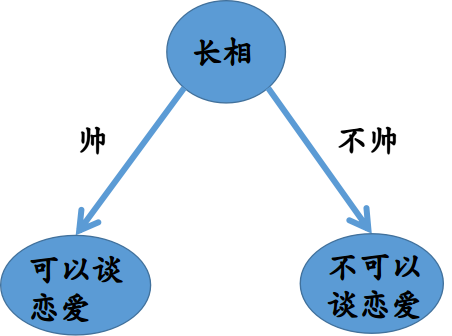如果你要求长得帅、皮肤白、身高180、均分90+、会打篮球的才可以和你谈恋爱，那可就要精挑细选了，你心目中
的决策树就会有很多层。类似这样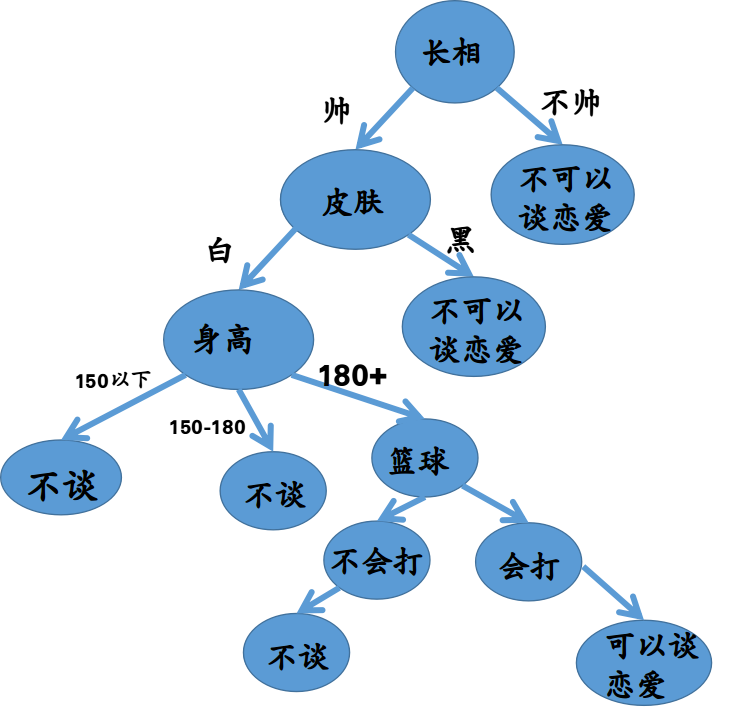看到这里我们应该大概知道决策树是怎么一回事了，但是真正用决策树的这种思想和算法去进行分类，我们用计算机
是怎样编程的呢？我们进行下一阶段的内容
上面举的例子只是帮助我们更直观的理解决策树的原理，但现实中绝对不会像例子中那么简单，需要考虑的不仅仅表面上
的客观条件，当然还要看对方能不能和你合得来，这个就比较复杂了，性格呀、感觉呀、爱情啊、好多变量是无法量化
的，所以上面的例子大家不要认真，千万别去问你心目中的女神找男朋友的决策树是怎样建立的，如果你问了，我敢保证，
你应该是不能谈恋爱的那个集合中的一个。
算法的数学原理
DecisionTree算法没有过多的数学原理，如果说有的话，就是在划分选择那里不同的划分选择会有不同形式的计算
方式。。决策树中的主要数学知识源于信息论理论。就是著名的科学家香农提出并作出重要贡献的信息论。
划分选择

信息增益(ID3算法)
信息熵是度量样本集合纯度最常用的一种指标。源于信息论，如果学过信息论的同学就很容易理解了，没有学过
信息论的同学可以借一本信息论基础的书籍都会有介绍。有些教程会说如果对数学原理不是很感兴趣，或者不能
理解数学公式的话可以跳过这一节，但是我不建议这样做，其实所有的知识都是由一个一个知识点组成的，特别
难的问题可能只是它的知识点比较多，这个时候不要逃避，去攻克一个又一个的小知识点我们才能有所成长。比如
这里的信息熵我们可以花上两个小时读一下信息论的知识，就会对整个决策树算法有了不同的理解方式，如果总是
逃避自己没有学过的知识那进步是非常缓慢的，我们要对未知的知识怀有挑战心，而不是畏惧心。这不仅是我给大
家的建议，同时也是给我自己的督促，大部分的时候我也会畏惧，我也会懒惰，所以遇到新知识要有征服的欲望而
不是逃跑，这对我们正在学习任何知识都是必要的。
闲话少说，回到信息熵。
信息论中一个离散型随机变量X的熵定义为H(X)=−∑x∈Xp(x)log2p(x)H(X)=-\sum_{x\in X}p(x)log_{2}p(x)p(x)p(x)表示随机变量X取值为x的概率。信息熵就是信息论中的离散平均自信息量。
假定当前样本集合U中第k类样本所占总样本树的比例为pkp_k, 则集合U的信息量为H(U)=−∑k=1npklog2pkH(U)=-\sum_{k=1}^n p_{k}log_{2}p_k
U中的类别越多代表需要确定某种类型所需要的信息量就会越多，也就是H(U)也会越大，这就是信息量的直观理解
假定某一离散属性用A表示，假设A表示的是人的身高段位，A可能的取值4个(认为划分)，假设有分别是(a1,a2,a3,a4)(a_1, a_2, a_3, a_4),分别表示150以下、150-170、170-180、180以上。若使用属性A对集合U进行划分，那么就会产生4个分支节点，
第i个分支节点包含U中样本AiA^i个，这AiA^i个样本组成了一个新的集合计为UiU^i。我们可以根据上面计算
信息熵的公式计算出UiU^i的信息量，怎么计算呢？假设身高在180以上的集合也就是U4U^4总共有20人，其中女
神中意的有2个，不中意的有18个，那么该集合按照中意这个标准的随机变量获得的信息量就是−(220log2220+1820log21820)-(\frac{2}{20}log_{2}\frac{2}{20}+\frac{18}{20}log_{2}\frac{18}{20})
同样的也可以求出其他分支集合的信息熵。
于是可计算出用属性A对样本U进行划分所获得信息增益(Information gain)
定义如下IG(U,A)=H(U)−∑i=14∣Ui∣∣U∣H(Ui)IG(U,A)=H(U)-\sum_{i=1}^{4}\frac{|U^i|}{|U|}H(U^i)
其中∣Ui∣|U^i|表示属于第i个分支中的样本数。一般而言，IG越大，意味着使用属性A进行划分获得的划分效果就越
好，因此我们可用信息增益来进行决策树的划分属性选择，著名的ID3决策树学习算法就是以信息争议为准则来选择
划分属性。

信息增益率(C4.5)
实际上，信息增益准则对可取值数目较多的属性有所偏好，为减少这种票好可能带来的不利影响，可以不直接使用
信息增益，而是使用信息增益率来选择最优的划分属性。
信息增益率(Information gain ratio)的定义如下：IGR(U,A)=IG(U,a)IV(A)IGR(U,A)=\frac{IG(U,a)}{IV(A)}
其中IV(A)=−∑i=1n∣Ui∣∣U∣log2∣Ui∣∣U∣IV(A)=-\sum_{i=1}^{n}\frac{|U^i|}{|U|}log_{2}\frac{|U^i|}{|U|}
称为属性A的固有值，属性A的可能取值数目越多，则IV(A)就会越大。增益率准则不是直接选取增益率最大的候选划分
属性，而是使用一种启发式先从候选划分属性中找到信息怎以高于平均水平的属性，再从中选择增益率最高的。

基尼指数(CART)
CART决策树使用了基尼指数来选择划分属性。基尼系数是1943年美国经济学家阿尔伯特·赫希曼根据洛伦兹曲线所定义的
判断收入分配公平程度的指标。基尼系数是比例数值，在0和1之间，是国际上用来综合考察居民内部收入分配差异状况的
一个重要分析指标。关于这部分内容可以参考Classification_and_Regression_Trees
这本书里有详细介绍，这里不做为重点讨论。

总之，上述三种算法的整体思想是一样的，就是通过划分属性来一步一步构建决策树，不同的地方在于划分的方法有所差异，我们在编程序过程中就可以根据不同的划分标准使用不同的函数。但整体的思路不变。
剪枝处理
剪枝是决策树学习算法解决“过拟合”问题的主要手段。在决策树学习中，也就是决策树的构建过程中，为了尽量
正确分类训练样本，结点划分过程将不断重复，有时候会造成分支过多，这时就是过拟合了，在神经网络学习过程
中可以通过提前结束训练或正则化方法来解决过拟合问题，在决策树学习中通常采用剪枝操作。剪枝操作又分为两种情况：预剪枝和后剪枝。下面分别阐述这两种方法。

预剪枝
预剪枝是指在决策树生成过程中，对每个节点在划分前先进行估计，若当前节点的划分不能带来决策树泛化能力的
提升，则停止划分，将当前节点标记为叶子节点。如何判断泛化性能提升呢？可以利用机器学习算法的评估方法(如
留出法)对剪枝前和剪枝后的性能进行评估，如果剪枝可以提高学习器的泛化性能，那么就进行剪枝操作，否则不进
行剪枝操作。
后剪枝
后剪枝先从训练集中生成一棵完整的决策树，然后分别考虑各个节点，如果去掉该节点后的决策树泛化能力更强那就
将此节点去掉即进行了剪枝操作，反之不进行剪枝操作。
以一个实例来进行讲解算法
C++实现
经过上面的原理介绍和细节的探讨估计大家已经指导决策树是怎样进行目标分类的了。
这里面我构建了一个DecisionTree的类，
DecisionTree说明文档
class DedisionTree
{
public:
struct Attr    //每一列的属性
{
int colIndex;
string Attribute;
int typeNum;   //属性取值的个数
vector<string> AttributeValue;
map<string, unsigned char>  typeMap; //属性取值对应的整数值;
};
struct TreeNode
{   //节点信息
string Attribute;   //此节点对应的属性
bool LeafNode; //如果是叶子节点，此值反映分类结果。 //其他情况都是0;
vector<TreeNode*> children; //孩子节点的地址。
map<string, TreeNode*> AttributeLinkChildren;
//属性指向孩子节点，也就是对应的树形结构中的树枝
};
//attributes
cv::Mat trainDataMat;
vector<vector<string>> predictedDataMat;
//MatInfo trainMatrixInfo;
TreeNode *root;  //根节点
vector<Attr> vectorAttr; //存储所有的矩阵信息，但不存储矩阵。

//functions
DecisionTree();    //默认构造函数
int ReadTrainDataFile(string fileAddress);  //数据预处理
TreeNode* BuildTree(cv::Mat &data, vector<Attr> &dataAttr, string AlgorithmName);
// 指定是哪种算法
vector<vector<string>> ReadPredictedDataFile(string fileAddreess);
vector<string> Predicted(TreeNode* root, vector<vector<string>> &pData);
//返回值为int类型表示数据的分类。
～DecisionTree();
}

TreeNode
TreeNode 结构体用来存储树的节点信息， Attribute 是std::string类型的数据用来存储当前节点的属性名称，如果该节点是中间节点(分支节点)，那么该值就是对应的属性名称，如果该节点是叶子节点，那么该值就是对应的分类结果。
**Attr **
Attr 结构体用来存储每一种属性的信息，包括这种属性有多少种不同的离散值,如下表所示表示根据不同的信息得出是否去打高尔夫的简单数据，以第一列数据为例，第一列表示天气属性，那么这一列信息对应着一个Attr结构，Attribute在这里的值就是Outlook，LeafNode及是标志，是否为叶子节点，如果是叶子节点，那么Attr存储的是最后一行分类的某个结果，比如说yes，children存储的是孩子节点的地址，AttributeLinkChildren存储的是孩子节点和属性值的映射关系，仍然以第一列数据为例，如果Outlook的结果是sunny那么此时指向sunny对应的叶子节点的地址。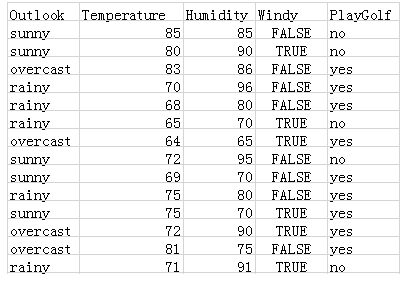trainDataMat
cv::Mat trainDataMat 存储训练集的数据，此结构由函数ReadTrainDataFile()生成
predictedDataMatvector<vector<string> > predictedDataMat存储的是待预测的数据矩阵，此矩阵由vector<vector<string>> ReadPredictedDataFile()函数生成
root
TreeNode *root 该属性存储决策树的根节点地址
vectorAttrvector<Attr> vectorAttr; 存储所有的属性信息
DecisionTree() 默认构造函数
ReadTrainDataFile()int ReadTrainDataFile(string fileAddress)
此函数执行读取训练数据的功能，输入值为待训练的数据集的地址，数据集的文件要求是必须为csv文件，关于csv文件参考百度百科 并且第一行的属性名称行是不能省略的，如下图的红色部分。此函数内部运行结束直接生成trainDataMat和vectorAttr数据，这两个数据在后面的操作中非常重要。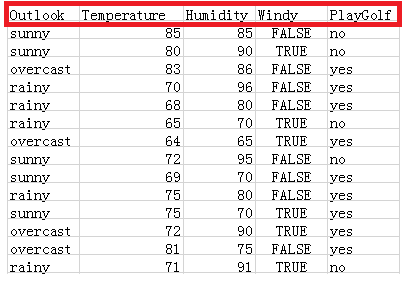BuildTree()TreeNode* BuildTree(cv::Mat &data, vector<Attr> &dataAttr, string AlgorithmName)
此函数用于生成决策树，函数的返回值是决策树根节点的地址，需要将此值返回给root属性，留给后面的预测时使用。
data 是训练集数据，类型为OpenCV中的Mat类型，对应类中的trainDataMat
dataAttr 是训练数据的属性信息，对应类中的vectorAttr
AlgorithmName 是需要指定的决策树划分节点属性类型的方法，这个类中只支持ID3、C4_5、和Gini这三种方法。
ReadPredictedDataFile()vector<vector<string>> ReadPredictedDataFile(string fileAddreess);
此函数执行读取待预测数据信息的功能，函数输入为待预测的数据，输出为字符串格式的矩阵。同样该文件是csv文件格式，但和ReadTrainDataFile有所差别就是该数据集不需要第一行的属性信息，如果有第一行的属性信息程序可能会出错，同时也不需要最后一列的标签列，对应的该矩阵的列数一定比训练矩阵的列数少1，行数没有限制。此函数输入的文件格式如下图,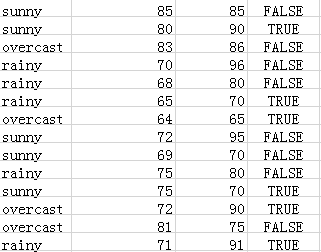Predicted()vector<string> Predicted(TreeNode* root, vector<vector<string>> &pData)
此函数执行预测的功能,函数的输入参数包括根节点地址root和预测数据矩阵pData。
Example
下面是简单的应用举例
输入数据说明
该类的两次输入分别是训练集和预测的测试集，并且都是csv格式的文件，此外目前算法只支持离散数据的分类，对于连续性数据不能使用，且每一列的属性个数不得多于255种，这是因为在程序设计中，每一列的数据个数的这个属性是采用uchar数据类型，所以不能多于255种，当然我们也可以根据自己的实际情况进行更改。程序的执行流程如下图所示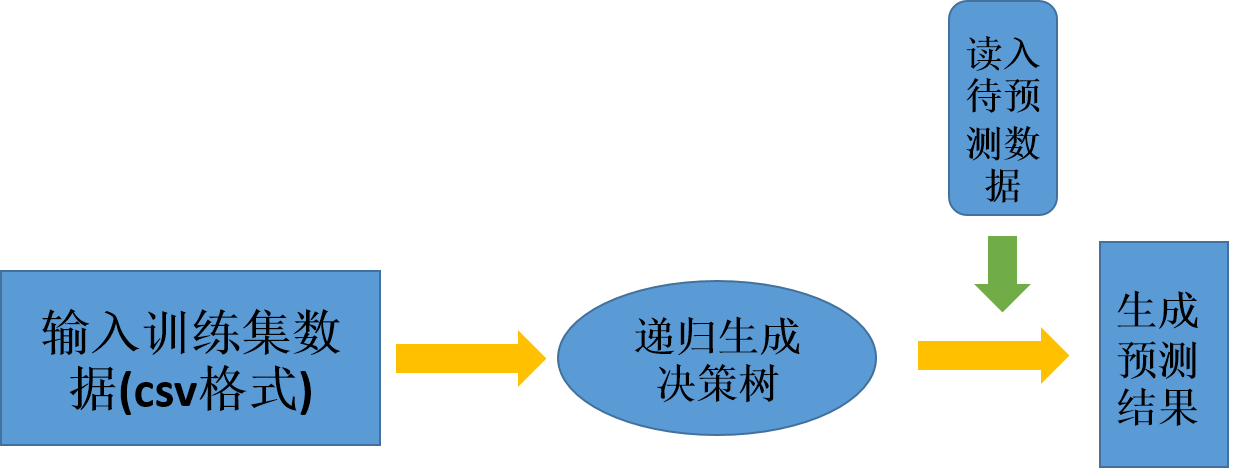//生成决策树对象
DecisionTree myDecisionTree;
//读取训练集数据并对训练数据进行操作
myDecisionTree.ReadTrainDataFile("data.csv");
//构建决策树,需要指明决策树划分的方法：ID3、C4.5、CART这三种
myDecisionTree.root = myDecisionTree.BuildTree(myDecisionTree.trainDataMat, myDecisionTree.vectorAttr, "ID3");
//
vector<string> result;
vector<vector<string>> predictedData;
//对待预测数据进行读取并进行处理
predictedData = myDecisionTree.ReadPredictedDataFile("pre.csv");
//预测结果
result = myDecisionTree.Predicted(myDecisionTree.root, predictedData);

在最后一步的预测中也可以不额外开辟 predictedData数据，因为在ReadPredictedDataFile()函数执行过程中已经备份一份数据到对象的predictedDataMat属性中，所以按照下面的方法会节省空间和时间result = myDecisionTree.Predicted(myDecisionTree.root, myDecisionTree.predictedDataMat);直接调用对象中的数据即可。
～DecisionTree()
～DecisionTree() 为构析函数
总结程序的优缺点
最开始的版本我刚刚开始学习C++，所以对于内存溢出的问题还不是特别敏感，所以在DecisionTree 类中动态开辟的内存空间都没有手动释放掉，主要是决策树的构建过程中开辟了许多堆空间，所以最终的版本需要加一个DestroyTree()函数将构建决策树过程中手动开辟的内存空间都释放掉。将内存泄漏的问题解决掉。还有程序中我遗留了四个问题 1.C4.5算法 2.CART算法 3.预剪枝 4. 后剪枝 这4个部分我还没有完成，我会慢慢完善所有功能，目前的版本只支持了ID3算法，其他算法也预留了函数位置，但是函数是空的，在调用过程中肯定会出错。
程序运行实例
#include "DecisionTree.hpp"
int main()
{
string fileAddress;
cout << "请输入文件的地址：";
cin >> fileAddress;
//cout << sizeof(unsigned int) << endl;
DecisionTree myDecisionTree;
myDecisionTree.ReadTrainDataFile(fileAddress);
myDecisionTree.root = myDecisionTree.BuildTree(myDecisionTree.trainDataMat, myDecisionTree.vectorAttr, "ID3");

//查看经过处理的样本矩阵
// cout << myDecisionTree.dataMat << endl;
cv::FileStorage fs("out.yml", cv::FileStorage::WRITE);
int t = int(myDecisionTree.vectorAttr.size());
for (int i = 0; i < t; i++)
{
fs << "Attribute" << myDecisionTree.vectorAttr[i].Attribute;
fs << "typeNum" << myDecisionTree.vectorAttr[i].typeNum;
// fs << "AttribureValue";
for (int j = 0; j < myDecisionTree.vectorAttr[i].AttributeValue.size(); j++)
{
fs << "value "<< myDecisionTree.vectorAttr[i].AttributeValue[j];
}
}
fs << "dataMat" << myDecisionTree.trainDataMat;
fs.release();

//进行预测
vector<string> result;
vector<vector<string>> predictedData;
predictedData = myDecisionTree.ReadPredictedDataFile("pre.csv");
result = myDecisionTree.Predicted(myDecisionTree.root, predictedData);
cout << "预测的结果如下：" << endl;
for (int i = 0; i < result.size(); i++)
{
cout << result[i] << endl;
}
system("pause");
return 0;
}

DecisionTree源代码
如果你是刚刚接触C++也不懂文件的依赖关系，也不懂的怎么用makefile编译，仅仅是想调用一下这个Decision用
一下的话，我建议你可以把我源代码中的DecisionTree的头文件和cpp文件放到你的工程里，然后include 头文件即可。
在下才疏学浅，很多地方还需要做的更好，如果有任何建议，请不吝赐教，我的昵称是PiggyGaGa，名为小猪嘎嘎。git同名。
欢迎留言。文章中有些内容借鉴周志华老师的西瓜书中的内容，仅当学习使用。
掌管天堂的空之王者，于地狱唱响荣光之歌！


展开全文C++
• 在学习机器学习决策树应用过程中，由于使用python2版本，遇到一些语法上的问题。 python2: allElectronicsData = open(r'C:\Users\IYQ\Desktop\Electronics.csv', 'rb') reader = csv.reader...
在学习机器学习决策树应用过程中，由于使用python2版本，遇到一些语法上的问题。
python2:

allElectronicsData = open(r'C:\Users\IYQ\Desktop\Electronics.csv', 'rb')
reader  = csv.reader(allElectronicsData)
headers = reader.next()
print(headers)

reader.next()报错：
AttributeError: '_csv.reader' object has no attribute 'next'

rb报错：
_csv.Error: iterator should return strings, not bytes (did you open the file in text mode?)

Electronics.csv文件格式报错：
UnicodeDecodeError: 'gbk' codec can't decode byte 0xff in position 0: illegal multibyte sequence

解决：在python3中已把reader.next()改成reader.__next__()，注意是两个下划线。同时把rb改成r。关于原始数据csv文件，保存编码格式为ANSI

python3:
allElectronicsData = open(r'C:\Users\IYQ\Desktop\Electronics.csv', 'r')
reader  = csv.reader(allElectronicsData)
headers = reader.__next__()
print(headers)


展开全文python csv
• 决策树是一种非常常用的机器学习算法，可以应用于分类和回归中，其中比较著名的有三种：ID3、C4.5和Cart算法。对于前两种只能针对分类，即离散数据集，且可以是多叉分类树；最后一种CART算法是分类决策树，既可以...
• 在如今机器学习领域，树可以说是最为重要的模型，在提高模型的准确率上有巨大威力的XGboost 都是基于树模型的，随机森林（包括多个决策树的分类器）也是基于树模型。 树模型 决策树，顾名思义就是和树一样，决策...信息增益 Gini系数
• 昨天的推送机器学习：对决策树剪枝，分析了决策树需要剪枝，今天再就这个话题，借助 sklearn 进一步分析决策树分类和回归时过拟合发生后，该如何解决的问题。 上周推送的机器学习：谈谈决策树，介绍了利用...
• 1. 什么是决策树/判定树（decision tree)?    判定树是一个类似于流程图的树结构：其中，每个内部结点表示在一个属性上的测试，每个分支...2. 机器学习中分类方法中的一个重要算法 3. 构造决策树的基本算法
• 运用python进行决策树分类预测。数据采用鸢尾花数据。（我也是python新手，将视频中的代码敲下来自练习消化。） # -*- coding: utf-8 -*- import numpy as np import pandas as pd from sklearn.datasets import ...python
•python 分类算法
• 决策树中的主要数学知识源于信息论理论。就是著名的科学家香农提出并作出重要贡献的信息论。 划分选择 信息增益(ID3算法) 信息熵是度量样本集合纯度最常用的一种指标。源于信息论，如果学过信息论的同学就很容易...
• 决策树（decision tree）是一个类似于流程图的树结构：其中，每个内部结点表示在一个属性上的测试，每个分支代表一个属性输出，而每个树叶结点代表类或类分布。树的最顶层是根结点。   熵（entropy）概念： ...深度学习
• 决策树算法是机器学习中的监督学习算法，决策树算法是一种逼近离散函数值的方法。它是一种典型的分类方法，首先对数据进行处理，利用归纳算法生成可读的规则和决策树，然后使用决策对新数据进行分析，其实就是通过一...算法 人工智能
• 此篇报告主要是对数据处理方面进行的一定个人总结和观点阐述，利用机器学习的方法对海量信息进行数据挖掘和统计分析，旨在于IT管理，企业可以将实时数据流分析和历史相关数据相结合，加深大数据对重要用户的洞察力。...pyhon 图像分析 PIL
• 很久没有更新博客了，一直以为自己默默无闻，... 今天就分享一下，我在研究学习 机器学习-决策树 这一部分知识时，遇到导出决策树，有时不支持中文。今天总算研究完整，实现了导出PDF文件、PNG图片都支持中文的方案...python 可视化
• 决策树作为一种分类算法，由于其强解释性与低学习成本，而广受欢迎，本篇文章仅从理论层面解释该算法的实现逻辑与数学推导过程。  说起决策树，离不开对信息熵的理解，该词来源于信息论，信息熵这个词对于我们来说...
• 1. 决策树的优势决策树作为处理分类问题最经常被用到的算法，它有着优于其他机器学习算法的简明性特征，即无需了解机器学习的原理知识也能够轻松的了解这个算法是如何工作的。2.决策树的构造 (1). 找到决定性的特征...python 算法
• 作者 | Prashant Gupta译者 | AI100（rgznai100）在实际生活中，树的类比如影随形。事实证明，树形结构对于...数据挖掘领域经常会用决策树来搜寻给定问题的解决策略，机器学习领域同样会广泛用到这一方法。这将会是...
• 标签： 机器学习决策树信息增益基尼指数模型评估 2016-05-04 15:31 43984人阅读 评论(1) 收藏 举报  分类： 机器学习（10）  版权声明：本文为博主原创文章，未经博主允许不得转载。 目录(?)[+]  机器学习...
• 机器学习决策树的作用 决策树算法 (Decision Trees Algorithm) A decision tree is a tree-like structure or graph based on decisions and their possible consequences to a situation. In a decision tree, ...算法 人工智能 python...